Everyday Math

# Geometry Warmup - Angles and Lines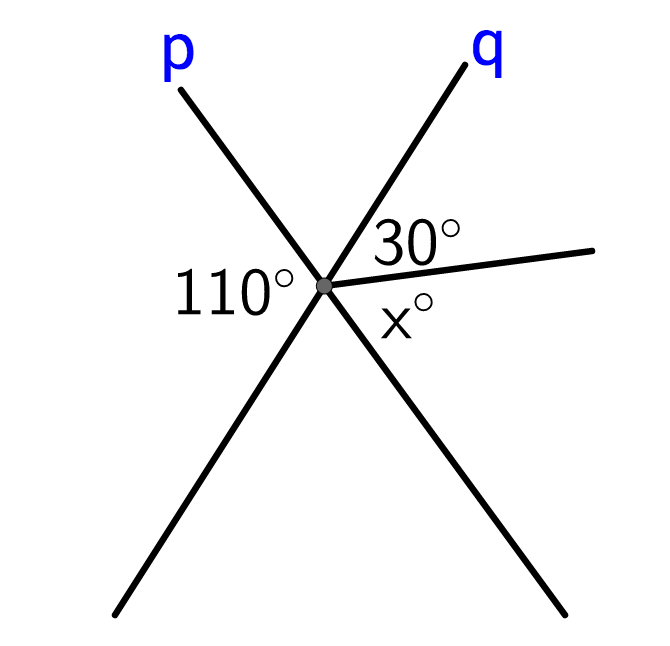Line $p$ intersects line $q.$ What is the value of $x?$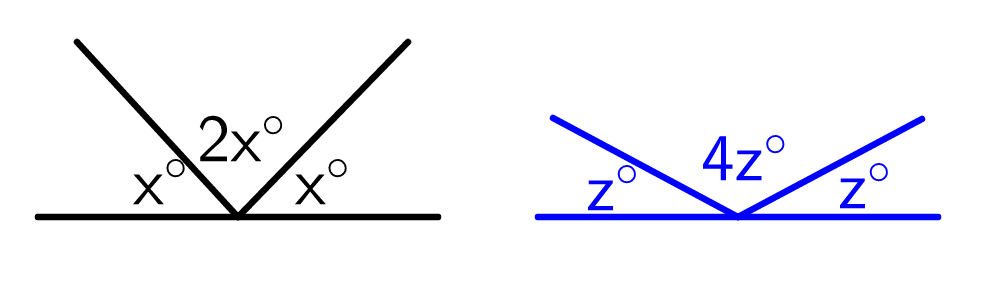$x$ is how many degrees larger than $z?$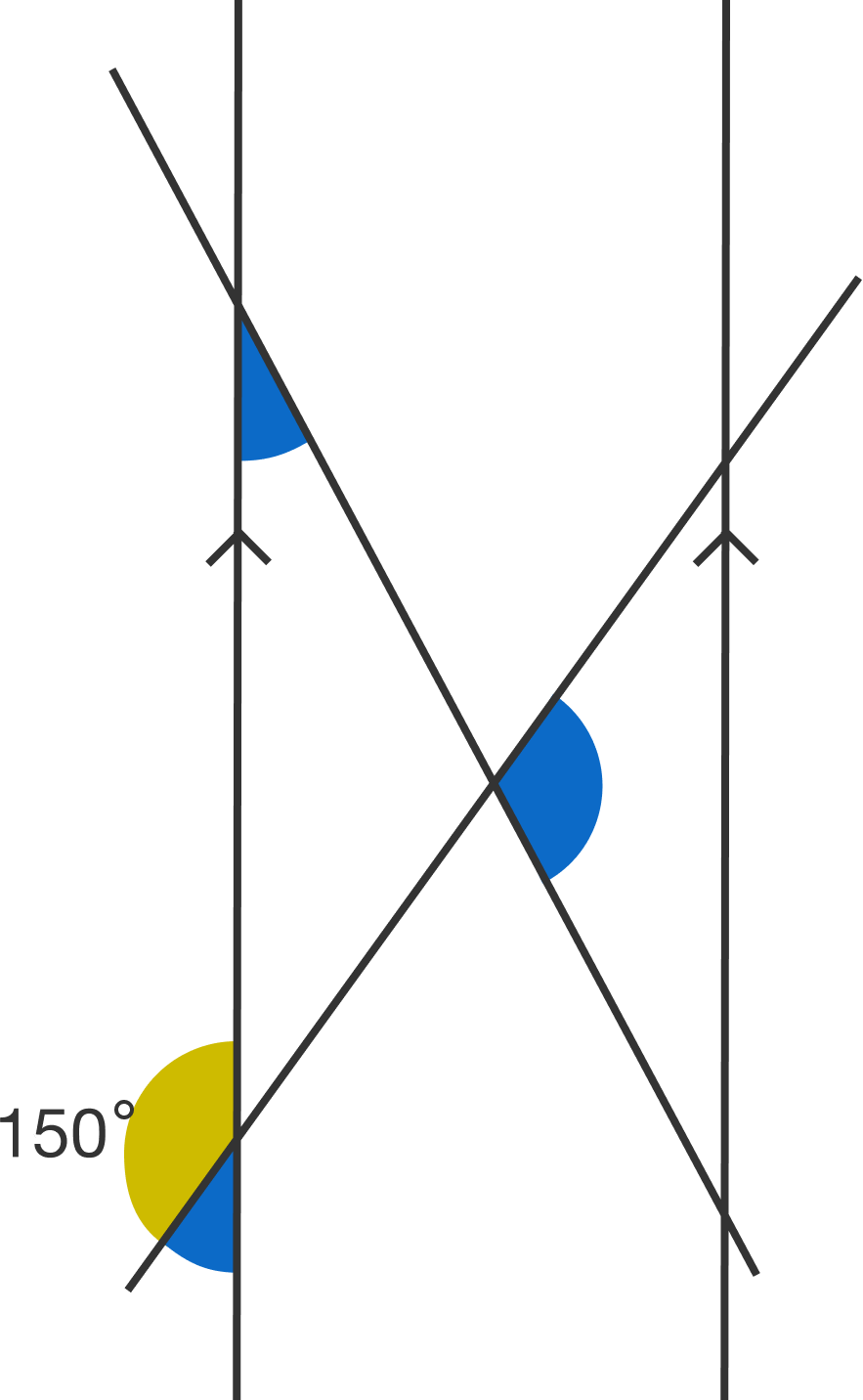The two vertical lines are perfectly parallel. What is the sum of the three blue angles?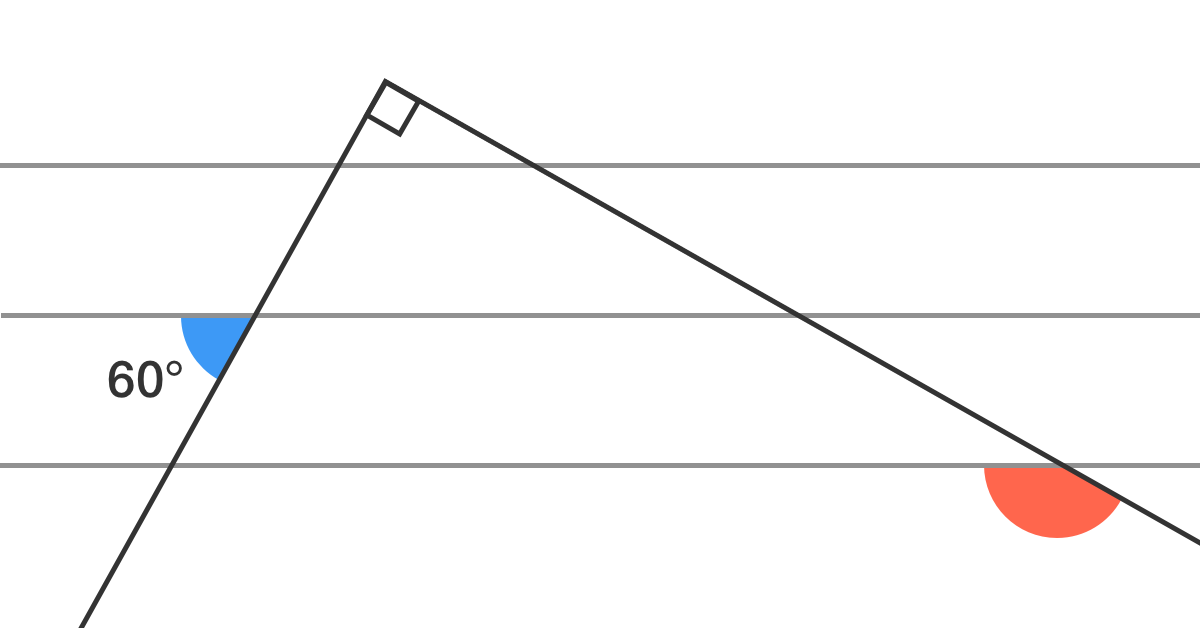Given that all three of the horizontal lines are parallel, what is the measurement of the red angle in degrees?

Note: The diagram is not drawn to scale.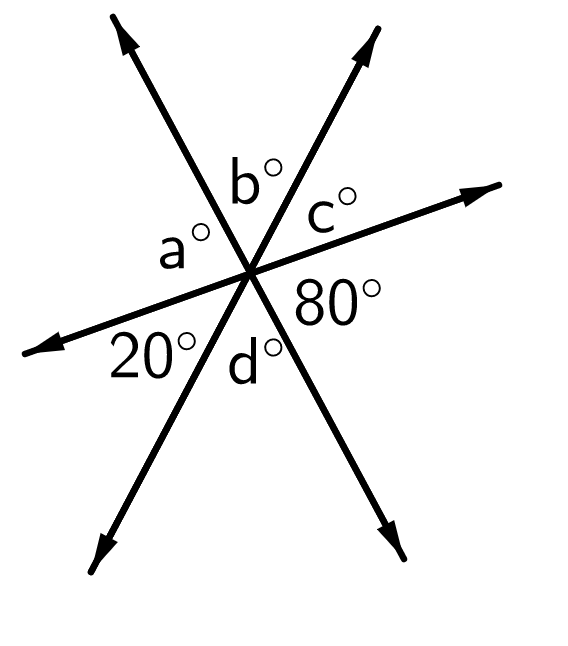What is the value of $a + b + c + d ?$

×